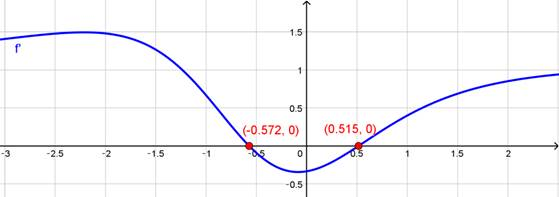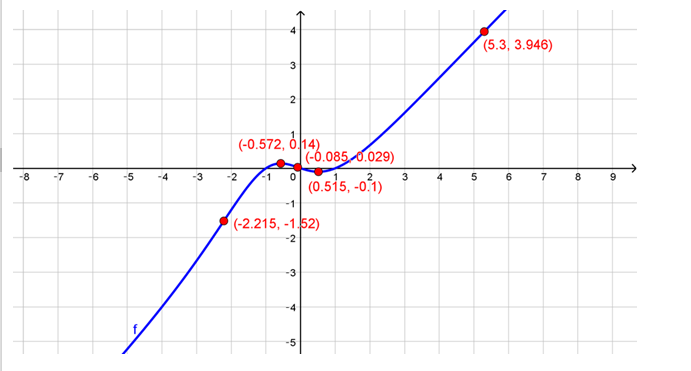# To graph and to estimate the intervals of increase, decrease and inflexion points.### Single Variable Calculus: Concepts...

4th Edition
James Stewart
Publisher: Cengage Learning
ISBN: 9781337687805### Single Variable Calculus: Concepts...

4th Edition
James Stewart
Publisher: Cengage Learning
ISBN: 9781337687805

#### Solutions

Chapter 4, Problem 16RE
To determine

## To graph and to estimate the intervals of increase, decrease and inflexion points.

Expert Solution

### Explanation of Solution

Given:

The given function is f(x)=x3xx2+x+3

Calculation:

f(x)=x3xx2+x+3

f'(x)=x4+2x3+10x23(x2+x+3)2f''(x)=6(x33x212x1)(x2+x+3)3From the above graph it can be estimated that f is increasing on (,0.57) and (0.52,)

Decreasing on (0.57,0.52)

There is a local maximum at f(0.57)=0.17

And a local minimum at f(0.52)=0.1The inflexion points are and (2.21,2.09) and (0.09,0.03)

### Have a homework question?

Subscribe to bartleby learn! Ask subject matter experts 30 homework questions each month. Plus, you’ll have access to millions of step-by-step textbook answers!